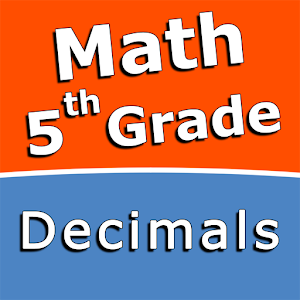Deutsch

Jeden Tag bieten wir KOSTENLOSE Android Apps und Spiele an die ihr sonst kaufen müsstet.\$2.99
ABGELAUFEN

# Android Giveaway of the Day - Decimals - Fifth grade Math skills

Practice and improve your math skills!
\$2.99 ABGELAUFEN
Benutzer Rating: 0 (0%) 0 (0%)

This giveaway offer has been expired. Decimals - Fifth grade Math skills is now available on the regular basis.

Intuitive interface powered by handwriting input and a choice of three fun and engaging mini games in addition to a regular math trainer mode make our app stand out from the crowd of generic math learning apps.

With Decimals - Fifth grade Math skills you can practice and improve the following math skills:
- Subtract decimal numbers
- Multiply a decimal by a power of ten
- Multiply a decimal by a one-digit whole number
- Multiply two decimal numbers
- Divide decimals by powers of ten
- Division with decimal quotients
- Divide decimals
- Convert decimals to fractions and mixed numbers
- Convert fractions and mixed numbers to decimals

Choose between four different modes:
- Math Trainer
- Math Shot mini-game
- Math Gravity mini-game
- Math Trick Shot mini-game

Sergey Malugin

Educational

1.0.0

11M

Everyone

4.0 and up

## Kommentare zum Decimals - Fifth grade Math skills

Thank you for voting!